# Power Flow Equation in Summation Form

Let us start from where we had left in the previous episode of this power flow analysis series and actually try to solve the power flow equation.

In that episode, we have seen the power flow equation in the compact form: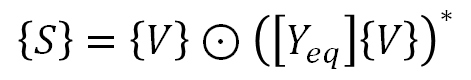Compact form of the power flow equation

To solve the power flow equation, it would be better to write the equation in the summation form (n is the number of nodes):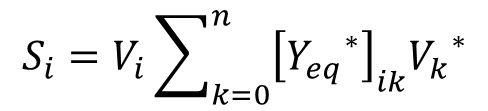Summation form of the power flow equation

If we look closely at the summation form of the equation, we can see that it has n separate equations. It also involves 2n separate variables, S_i and V_i for every node #i.

This means that we will need to set n variables as fixed to solve for the rest of the n unknown variables.

# Power and Voltage as Complex Variables

Ok, but we also need to remember that {S} and {V} are complex variables, and a complex variable contains 2 parts of information.

In rectangular coordinates the information is stored in the real and imaginary parts of the variable.

In polar coordinates it is stored in the magnitude and phase angle of the variable.

Usually, people write the complex power {S} in the rectangular coordinate form, so

where {P} is the real power and {Q} the reactive power.

On the other hand, people usually write the complex voltage {V} in the polar coordinate form, so

where {U} is the magnitude of voltage and {θ} the phase angle of voltage.

People use both forms of coordinates to write the node admittance [Y_eq], but in this video we will stick to the polar coordinate form, so

where [Y bar] is the magnitude of node admittance and {φ} the phase angle of node admittance.

So with the complex version of the summation form, we actually will have 2n separate equations and 4n variables, 2n of which must be held fixed in order to solve for the other 2n variables.

Here are the detailed equations for solving {P} {Q} {U} and {θ}: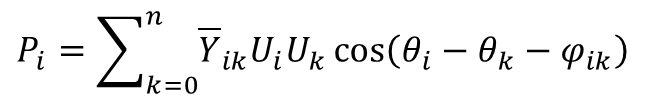Real part of the rectangular form of the power flow equation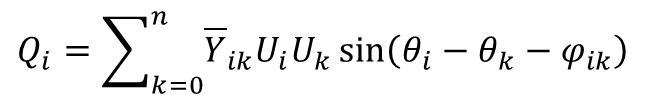Imaginary part of the rectangular form of the power flow equation

If for each node, 2 of these variables are given, then the remaining 2n unknown variables will form an exactly determined system of 2n equations.

# Types of Nodes in a Power System

Depending on the known and unknown variables, we can categorize the nodes in the power system into several types of buses. Below are the 3 most common types:

1. PQ bus: at this type of node, the real and reactive power are given, and we solve for U and θ.
2. PU bus: at this type of node, the real power P and the voltage magnitude U are given, and we solve for the reactive power Q and the voltage phase angle θ.
3. Slack bus: at this type of node the magnitude and phase angle of voltage are known. The real and reactive power are unknown, but it is not relevant.

Note that while these are the most common types of nodes, other types of nodes do exist.

For instance, the demand load of a node may have a constant P / Q ratio (this ratio is usually called the power factor) and a fixed value of U.

For demand loads with constant impedance, the corresponding node must satisfy the constraint:

In any case, we will always need 2n equations additional to the complex form of the power flow equation to solve the system.

# Examples of types of Nodes

Let’s take a look at what are usually modeled by each type of nodes.

1. We usually model demand load and variable renewable energy without batteries with PQ buses.
2. Meanwhile, conventional generators and dispatchable renewable energy are usually modeled as PU buses.
3. Finally, grid-forming inverters with batteries are examples for slack buses.

Please note that the purpose of this chart is not to provide a clear cut categorization; the type of bus of a node depends on the controllability of the devices connected on the node.

For example, VRE with grid support inverters can be viewed as a PU bus to some extent; meanwhile when a conventional generator reaches its reactive power limit, it can turn into a PQ bus.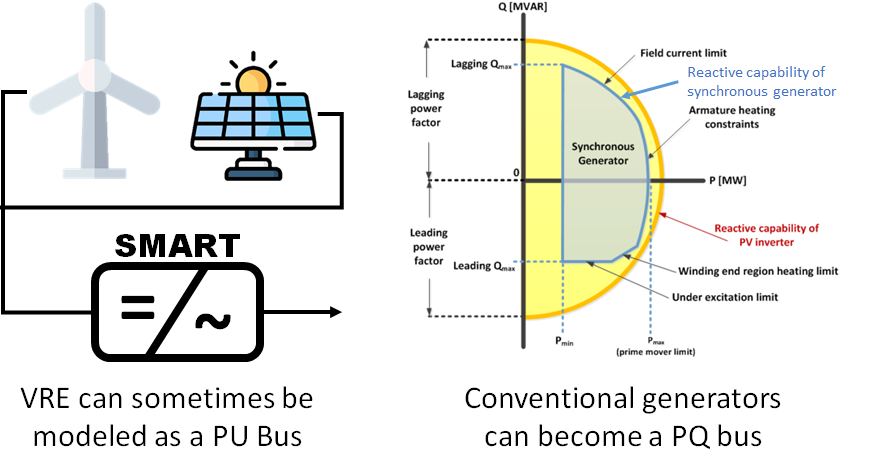The graph on the right is adapted from figure 39 of a NREL report in March 2017: “Demonstration of Essential Reliability Services by a 300-MWSolar Photovoltaic Power Plant”. Read the report from this link.

# Procedure of the Newton-Raphson Method

To demonstrate how the Newton-Raphson method works, assume (for the sake of simplicity) all the nodes in the power system are PQ buses, and we solve for the voltage magnitude and phase angle for each node.

We do so by starting with an initial guess of U and theta, which we just call them {U}_g and {θ}_g.

Next, let us inert {U}_g and {θ}_g into the power flow equation; this will result in the first guess of {P} and {Q}, denoted as {P}_g and {Q}_g.

Now we can update {U}_g and {θ}_g by the following rule:

Where J is the Jacobian matrix:

We then repeat this procedure until {U}_g and {θ}_g converge to a fixed point (if that actually happens) or until we run a sufficient large amount of iterations.

# Calculation of the Jacobian Matrix

To calculation the Jacobian matrix, let us view the real and reactive power as functions of voltage magnitude and phase angle, and take the partial derivatives of them according to the summation form of the power flow equation.

We can therefore obtain the following equations for the partial derivatives:

Here the letter delta indicates the Kronecker-Delta function:

# Potential Problems of the Newton-Raphson Method

What are the problems when using Newton-Raphson method?

The first is that the final solution is sensitive to the initial guess. Therefore, there is no guarantee that the final solution converges to the high voltage solution.

Also, for each iteration the Jacobian matrix and its inverse must be re-calculated again; this increases the computational burden of the method.

In the next videos, we will discuss methods that deal with these shortcomings.

--

--## Tony Yen

A Taiwanese student who studied Renewable Energy in Freiburg. Now studying smart distribution grids / energy systems in Trondheim.